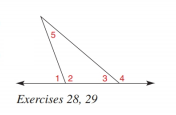Chapter 2.4, Problem 28E### Elementary Geometry for College St...

6th Edition
Daniel C. Alexander + 1 other
ISBN: 9781285195698

#### Solutions

Chapter
Section### Elementary Geometry for College St...

6th Edition
Daniel C. Alexander + 1 other
ISBN: 9781285195698
Textbook Problem
1 views

#Given: m ∠ 1 = 8 ( x + 2 ) m ∠ 3 = 5 x − 3 m ∠ 5 = 5 ( x + 1 ) − 2 Find: x

To determine

To find:

The value of x.

Explanation

Given:

m1=8(x+2)m3=5x3

And,

m5=5(x+1)2

Figure (1)

Approach:

The measure of an exterior of a triangle is equal to the sum of the measures of two non-adjacent interior angles.

Calculation:

Equate the exterior angle of triangle to the sum of the measures of two non-adjacent interior angles.

m1=m5+m3

Substitute 8(x+2) for m1<

### Still sussing out bartleby?

Check out a sample textbook solution.

See a sample solution

#### The Solution to Your Study Problems

Bartleby provides explanations to thousands of textbook problems written by our experts, many with advanced degrees!

Get Started

#### Find the constants m and b in the linear function f(x) = mx + b such that f(0) = 2 and f(3) = 1.

Applied Calculus for the Managerial, Life, and Social Sciences: A Brief Approach

#### The graph at the right has equation:

Study Guide for Stewart's Multivariable Calculus, 8th

#### The absolute maximum value of f(x) = 6x − x2 on [1, 7] is: 3 9 76 −7

Study Guide for Stewart's Single Variable Calculus: Early Transcendentals, 8th